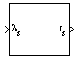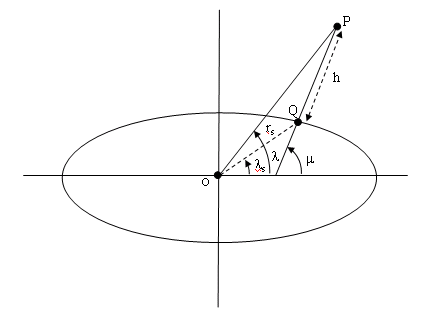Estimate radius of ellipsoid planet at geocentric latitude

• Library:
• Aerospace Blockset / Flight Parameters

•## Description

The Radius at Geocentric Latitude block estimates the radius $\left({r}_{s}\right)$ of an ellipsoid planet at a particular geocentric latitude $\left({\lambda }_{s}\right)$.The following equation estimates the ellipsoid radius $\left({r}_{s}\right)$ using flattening $\left(\overline{f}\right)$, geocentric latitude $\left({\overline{\lambda }}_{s}\right)$, and equatorial radius $\left(\overline{R}\right)$:

`${r}_{s}=\sqrt{\frac{{R}^{2}}{1+\left[1/{\left(1-f\right)}^{2}-1\right]{\mathrm{sin}}^{2}{\lambda }_{s}}}.$`

## Ports

### Input

expand all

Geocentric latitude, specified as a scalar or vector, in degrees.

Data Types: `double`

### Output

expand all

Radius of planet at geocentric latitude, returned as a scalar or vector, in the same units as flattening.

Data Types: `double`

## Parameters

expand all

Output units, specified as:

`Metric (MKS)` MetersMeters
`English` FeetFeet

#### Dependencies

To enable this parameter, set Planet model to `Earth (WGS84)`.

#### Programmatic Use

 Block Parameter: `units` Type: character vector Values: ```'Metric (MKS)'``` | `'English'` Default: ```'Metric (MKS)'```

Planet model to use, `Custom` or `Earth (WGS84)`.

#### Programmatic Use

 Block Parameter: `ptype` Type: character vector Values: ```'Earth (WGS84)'``` | `'Custom'` Default: ```'Earth (WGS84)'```

Flattening of the planet, specified as a double scalar.

#### Dependencies

To enable this parameter, set Planet model to `Custom`.

#### Programmatic Use

 Block Parameter: `F` Type: character vector Values: double scalar Default: `1/298.257223563`

Radius of the planet at its equator, in the same units as the desired units for ECEF position.

#### Dependencies

To enable this parameter, set Planet model to `Custom`.

#### Programmatic Use

 Block Parameter: `R` Type: character vector Values: double scalar Default: `6378137`

 Stevens, Brian L., Frank L. Lewis. Aircraft Control and Simulation, New York, John Wiley & Sons, 1992.

 Zipfel, Peter H., Modeling and Simulation of Aerospace Vehicle Dynamics. Second Edition. Reston, VA: AIAA Education Series, 2000.

## Support평가판 신청